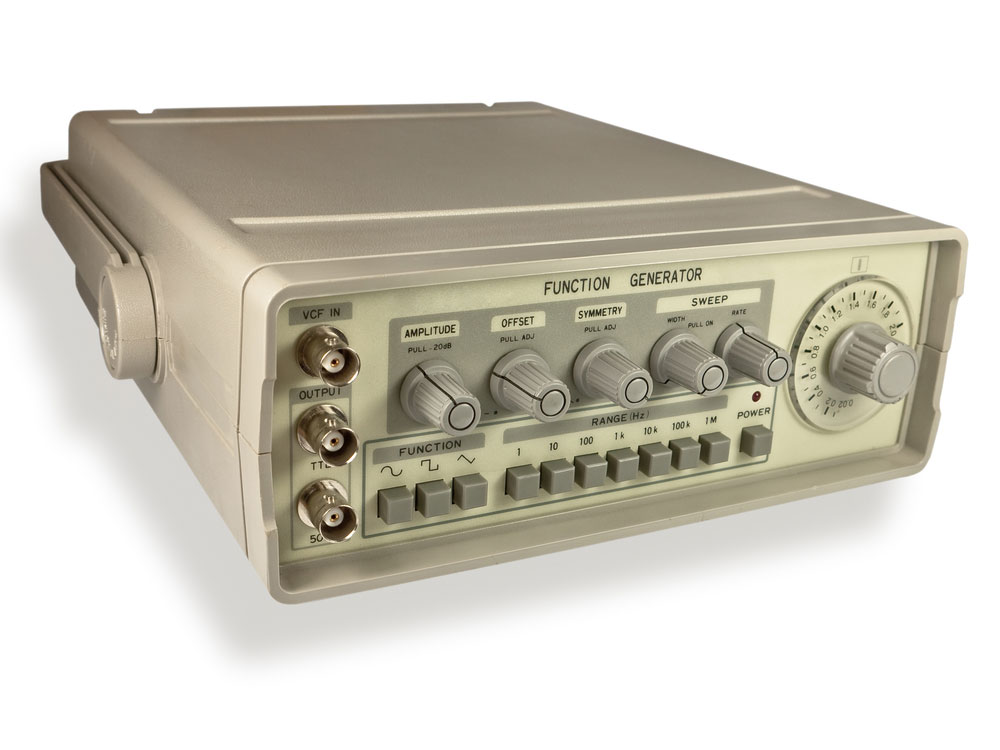Blog  /  555 Pulse Generator: Basics and Applications

# 555 Pulse Generator: Basics and Applications

Are you looking to produce positive pulses for your primary electronics circuit? Look no further than the 555 pulse generator.

But how does this adjustable pulse generator operate? We are going to explain it all explicitly. Also, we'll look at the electronic components that require the integrated circuit.

Let's get started!

## What is a 555 Pulse Generator?Signal Generator

A pulse generator is an integrated circuit capable of producing pulses, and it is common in timer circuits, pulse generators, and oscillators.

## How to make a 555 pulse generator Module?Old green analog oscilloscope display with pulse image.

In the assembly of this pulse generator, the cardinal component is the 555 timer IC. The IC is an astable multivibrator. Note that the pulse generator can produce an output pulse of 4Hz to 1.3KHz.

The generator produces positive pulses. Thus, you can use it in an electronic project that requires positive vibrations.

Also, you'll need an LED at the IC's output in assembly. It is helpful for visual indication of the output pulse of the system.

A potentiometer helps control the output frequency of the system. The ideal circuit voltage of this system is anything between 5V and 15V. Also, the circuit runs on a DC power supply.

### Circuit Component ListAn Electronic integrated circuit chip

A

• A 555 Timer IC
• A 10μF Capacitor
• A 1kΩ Resistor
• A 10kΩ Potentiometer

### Circuit Diagram

Here is the circuit layout of the IC 555 pulse generator555 pulse generator circuit diagram

Connect the components above as shown on the circuit diagram.

### How the circuit works

When you connect Pins 2 and 6, you set the timer to an astable mode.

The astable mode leads to two primary outcomes. First, the state prompts the retriggering of the timer. Consequently,  there will be the generation of a stream of pulses. In this case, the vibrations are PWM signals. And the timer will keep generating them as long as you have connected it to an input voltage.

Furthermore, note that pin 3 acts as the output pin. Therefore, changing the value of resistances and capacitances will alter the output pulse's frequency. Also, it will change the duty cycle of the square wave from the output pin.

Note that the capacitor in this circuit charges and discharges in every cycle. When the capacitor is charging, it leads to 'ON' times. On the other hand, capacitor discharge causes an 'OFF' time.

Note that it's possible to calculate the circuit's output frequency and duty cycle. The critical values in this calculation include the following:

Rx= 1kΩ

Ry= 10kΩ

First, we'll calculate the t1 value. It is the timer value when the capacitor charge is in the ON mode.

The circuit equation of the t1 value = 0.693 * (Rx + Ry) * C

#### When we substitute the values, the equation yields 76.23 milliseconds.

Next, we'll calculate the t2 value. The timer value is when the capacitor electric charge is in OFF mode.

The Equation for t2 value= 0.693 * Ry *C

When substituting the values, the solution to the equation is 69.3 milliseconds.

Next, we can calculate the timer's periodic (T) or pulse period with the two solutions.

T= t1 + t2 = 145.53 milliseconds

Also, we can evaluate the output frequency of the timer with the periodic time.

f = 1/T. Hence f = 6.871 Hertz.

## Applications

• Managing the speed of DC motors
• Generation of a square wave signal
• Generation of an adjustable wave for an MCU
• In stepper motor applications
• Useful in telecommunications for encoding purposes
• Adjustable pulse generation for circuits control

## 555 Pulse Generator Circuits

There are several types of 555 pulse generator circuits, and they are helpful in oscillators. Hence, we'll look at the various kinds of oscillators where they are applied.

### Simple 555 timer astable oscillator circuit

The circuit that we earlier described is what produces this pulse generator module. It is a primary circuit that forms the foundation of creating other courses. And we'll highlight them below. The average current in this circuit is 200mA maximum.

### High Power 555 Pulse GeneratorHigh Power 555 Pulse Generator Circuit Diagram.

It is ideal when you want a pulse oscillator for a high current. In this circuit, there are two primary components. First, there is the IC 555, which is the oscillator, and an LM350T. The latter is significant in increasing the current signals to 3 A.

### Pulse generator control frequency using digital ICPulse generator control frequency Circuit Diagram

You can also refer to this assemblage as an astable multivibrator circuit. It oscillates when it produces frequencies more significant than one cycle in a second. On the other hand, it is a time delay if it makes frequencies more petite than a cycle in one second.

Other standard circuits using IC 555 include the following:

1. The danger beep circuit using IC-555.
2. Tone Burst Generator using LM555.
3. Annoying high pitch noise generator using IC-555.

## Final Thoughts

As you might have noted, the pulse generator has a wide range of applications, and hence, there is a high likelihood that you'll need it for your electronics project. In a nutshell, we have expounded on all the critical aspects of the 555 pulse generator. And there you have it all.

The rule of thumb is ensuring you understand the circuitry, and we have illustrated this effectively using elaborate circuit diagrams. If you have a question about anything on the pulse generator, contact us